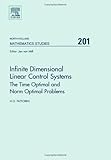Home FAQ Random quotes Contact

# Infinite Dimensional Linear Control Systems, Volume 201: The Time Optimal and Norm Optimal Problems (North-Holland Mathematics Studies)ISBN13: 9780444516329
ISBN: 0444516328
Binding: Hardcover
List Price: \$175.0
Publisher: North Holland
Published Date:
Edition 1
Pages: 332

For more than forty years, the equation y (t) = Ay(t) + u(t) in Banach spaces has been used as model for optimal control processes described by partial differential equations, in particular heat and diffusion processes. Many of the outstanding open problems, however, have remained open until recently, and some have never been solved. This book is a survey of all results know to the author, with emphasis on very recent results (1999 to date).
The book is restricted to linear equations and two particular problems (the time optimal problem, the norm optimal problem) which results in a more focused and concrete treatment. As experience shows, results on linear equations are the basis for the treatment of their semilinear counterparts, and techniques for the time and norm optimal problems can often be generalized to more general cost functionals.

The main object of this book is to be a state-of-the-art monograph on the theory of the time and norm optimal controls for y (t) = Ay(t) + u(t) that ends at the very latest frontier of research, with open problems and indications for future research.

Key features:

. Applications to optimal diffusion processes.
. Applications to optimal heat propagation processes.
. Modelling of optimal processes governed by partial
differential equations.
. Complete bibliography.
. Includes the latest research on the subject.
. Does not assume anything from the reader except
basic functional analysis.